# 西安空气质量数据的回归分析Regression Analysis of Air Quality Data in Xi’an

• 全文下载: PDF(2481KB)    PP.731-742   DOI: 10.12677/AAM.2018.76088
• 下载量: 513  浏览量: 2,092   科研立项经费支持

Air is the condition for human beings and organisms to survive, so clean air is especially important to people. However, in recent years, with the development of China’s industry and transportation industry, a large number of pollutants have been discharged into the air, the quality of air is getting worse and worse, and the problem of air quality has been paid more and more attention by the government and the public. In order to explore the close correlation between air quality and which pollutants and the relationship between them, this article selected Xi’an as an example to collect Xi’an air quality data from December 2013 to April 2018, mainly including air mass index (AQI) and PM2.5, PM10, SO2, CO, O3 and so on. R software was used for regression analysis. First, the air quality data of December 2013-2017 year June are used to model the model, and the model is tested. Then the data of April July 2107-2018 are used to predict the AQI index, in order to test the model.

1. 引言

2. 回归分析建模

2.1. 建立一般多元线性回归模型

1) 散点图

2) 拟合模型

3) 回归诊断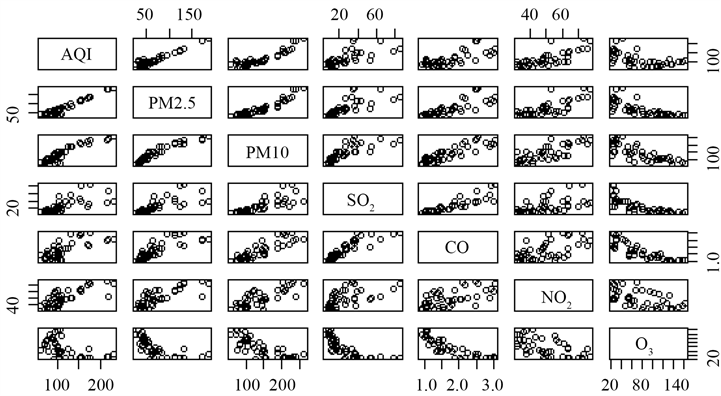Figure 1. Scatter plot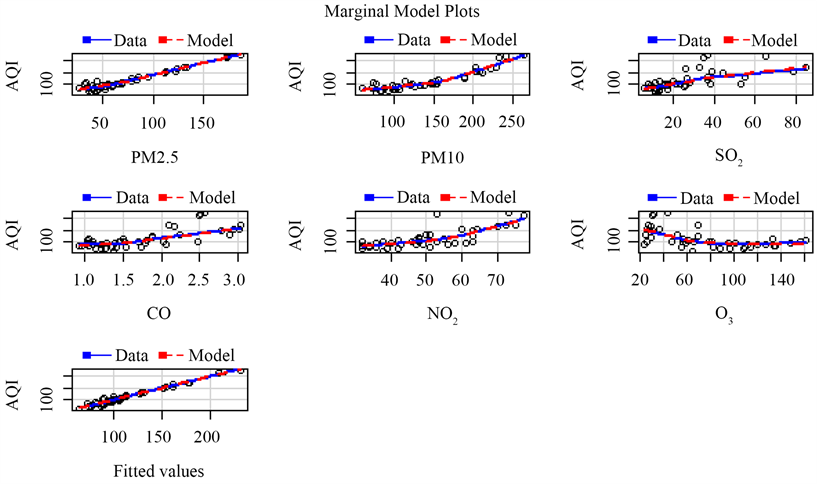Figure 2. Marginal model diagram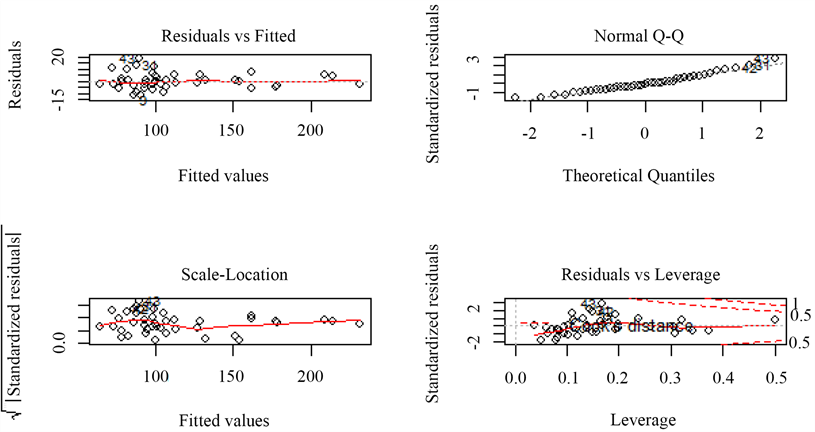Figure 3. Regression diagnosis chart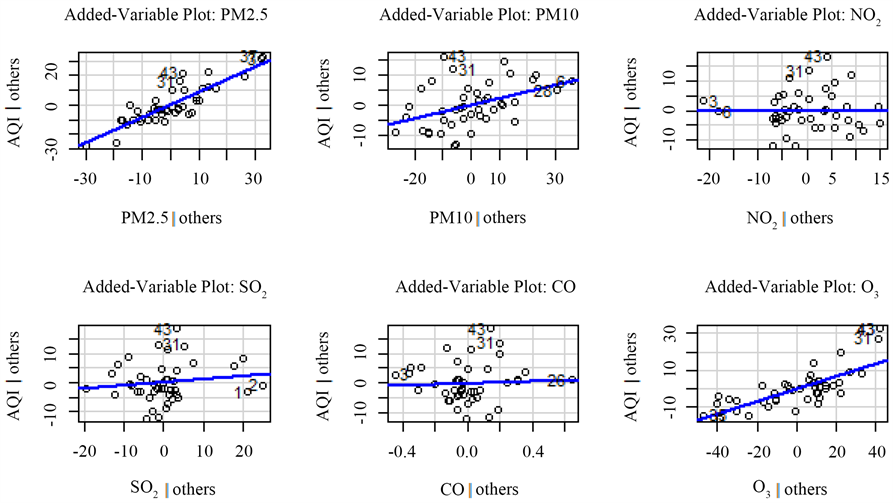2.2. 变换变量建模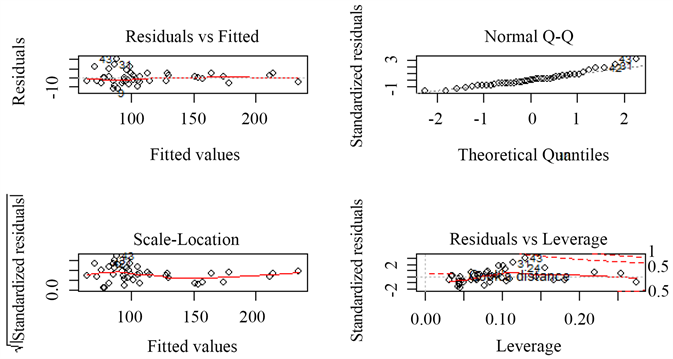Figure 5. Regression diagnosis chart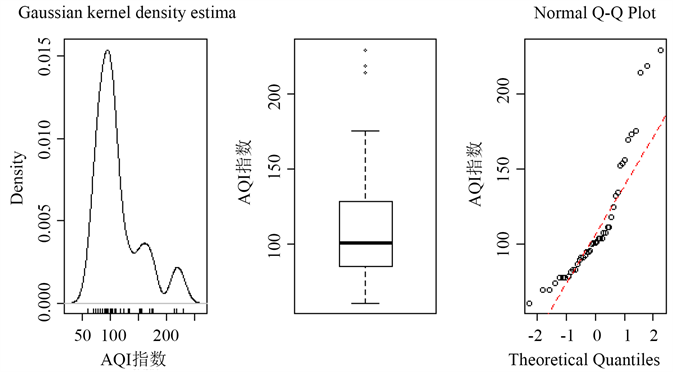Figure 6. AQI: normal distribution test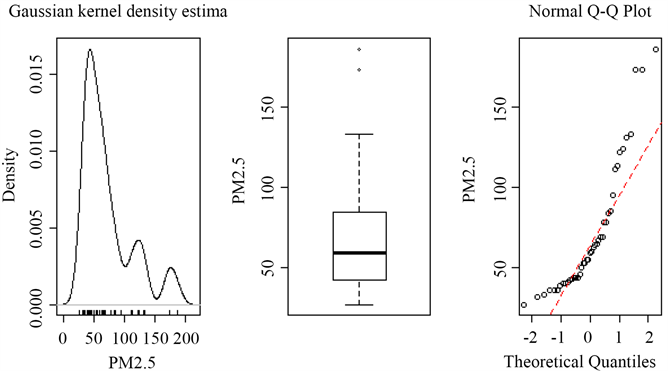Figure 7. PM2.5: normal distribution test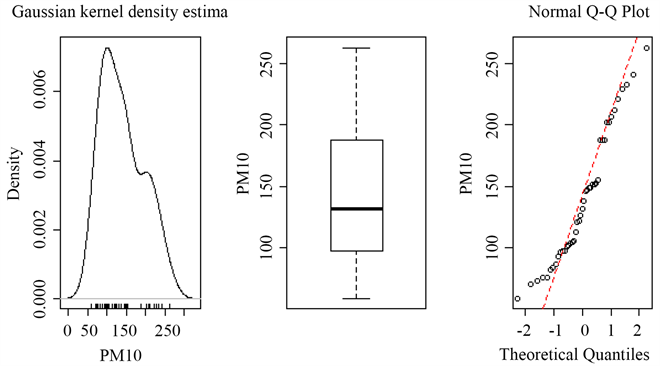Figure 8. PM10: normal distribution test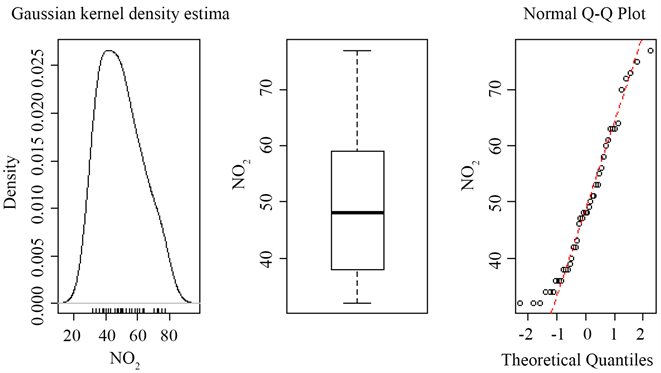Figure 9. NO2: normal distribution test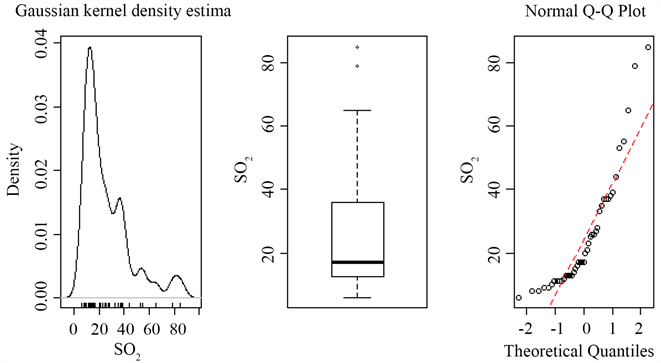Figure 10. SO2: normal distribution test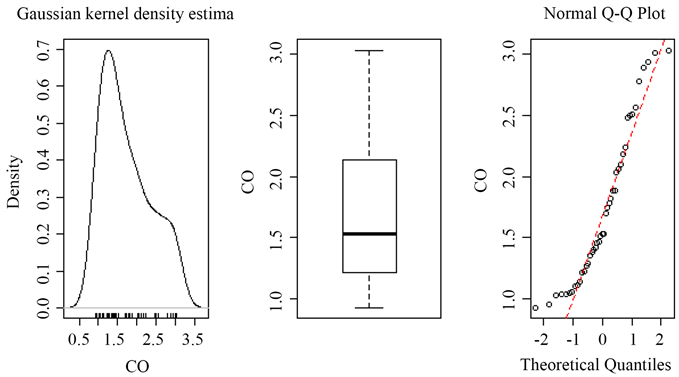Figure 11. CO: normal distribution test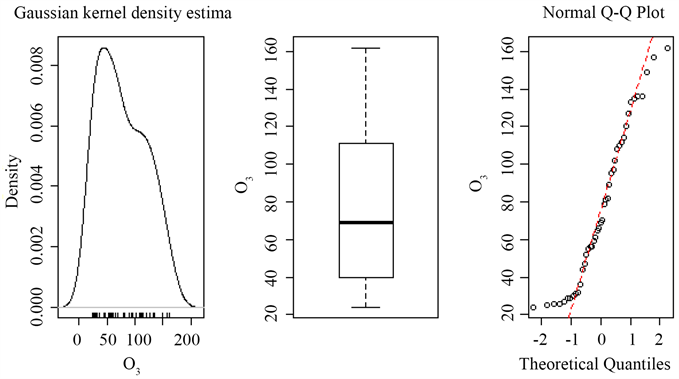Figure 12. O3: normal distribution test

tPM2.5 PM10 NO2 tSO2 tCO O3

28.211357 24.0693573.452873 10.288414 14.752069 6.674461Table 3. BOX-COX transformation resultsTable 5. Variable selection results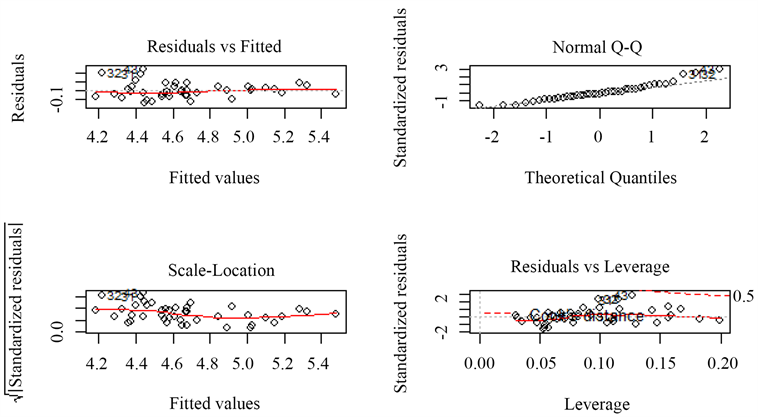Figure 13. Regression diagnosis chart

$\text{log}\left(\text{AQI}\right)=0.\text{3995}0\text{6log}\left(\text{PM2}.\text{5}\right)+0.00\text{3997PM1}0+0.00{\text{3511O}}_{\text{3}}+\text{2}.\text{187334}$

3. 预测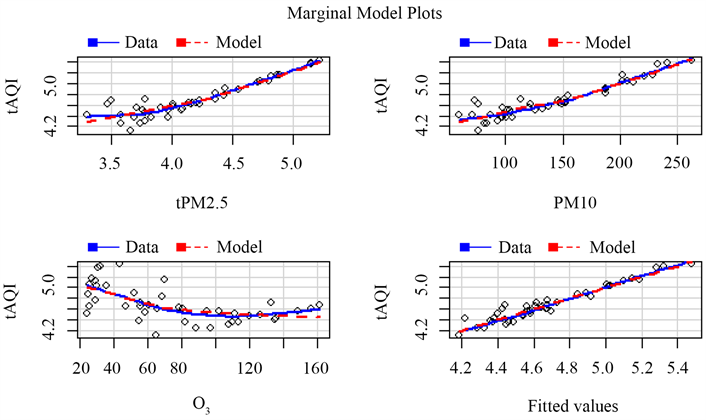Figure 14. Marginal model diagram

4. 总结

NOTES

*通讯作者。

  西安空气质量指数AQI-PM2.5月统计历史数据-中国空气质量在线监测分析平台历史数据[EB/OL]. https://www.aqistudy.cn/historydata/monthdata.php?city=%E8%A5%BF%E5%AE%89  李丹. 基于聚类分析和多元回归的空气质量的分析[D]: [硕士学位论文]. 天津: 南开大学数学科学学院, 2015.  李晓童, 夏明月, 林善冬. 基于Bootstrap方法对北京空气质量的回归分析[J]. 河北北方学院学报(自然科学版), 2014, 30 (4): 31-34.  肖正, 祁孟阳, 朱家明. 基于多元线性回归模型的合肥市空气质量实证分析[J]. 兰州文理学院学报(自然科学版), 2017, 31(4): 13-19.  Samprit Chatterjee, Ali S. Hadi. 例解回归分析[M]. 北京: 机械工业出版社, 2013.  Robert I. Kabacoff. R语言实战[M]. 北京: 人民邮电出版社, 2016.